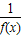• 试题检索 :
• 教材版本 :
• 课本 :
• 题型 :
• 难易度 :

①x，y∈R，若x2+y2=0，则x=0或y=0的否命题是假命题；
②函数y=3x+3-x(x＜0)的最小值为2；
③若函数f(x)=x3+ax2+2的图象关于点(1，0)对称，则a的值为-3；
④若f(x+2)+=0，则函数y=f(x)是以4为周期的周期函数；
⑤若(1+x)10=a+a1x+a2x2+…+a10x10，则a+a1+2a2+3a3+…+10a10=10×29其中真命题的序号是

“若x、y全为零，则xy=0”的否命题为

(1)命题“若q则p”与“若￢p则￢q”互为逆否命题；
(2)“am2＜bm2”是“a＜b”的充要条件；
(3)“矩形的两条对角线相等”的否命题是假命题；
(4)命题“∅⊆{1，2}”为真命题，其中正确的序号是
“若x≠a且x≠b，则x2-(a+b)x+ab≠0”的否命题